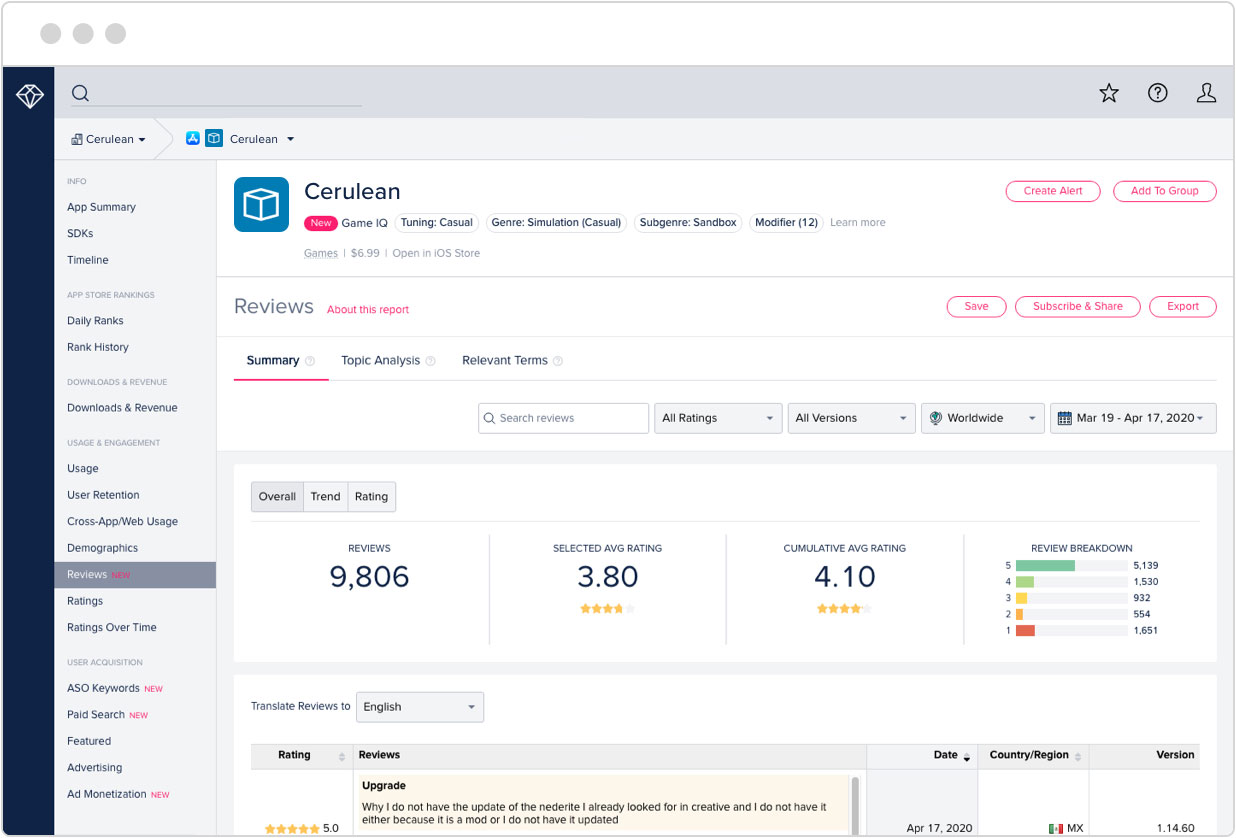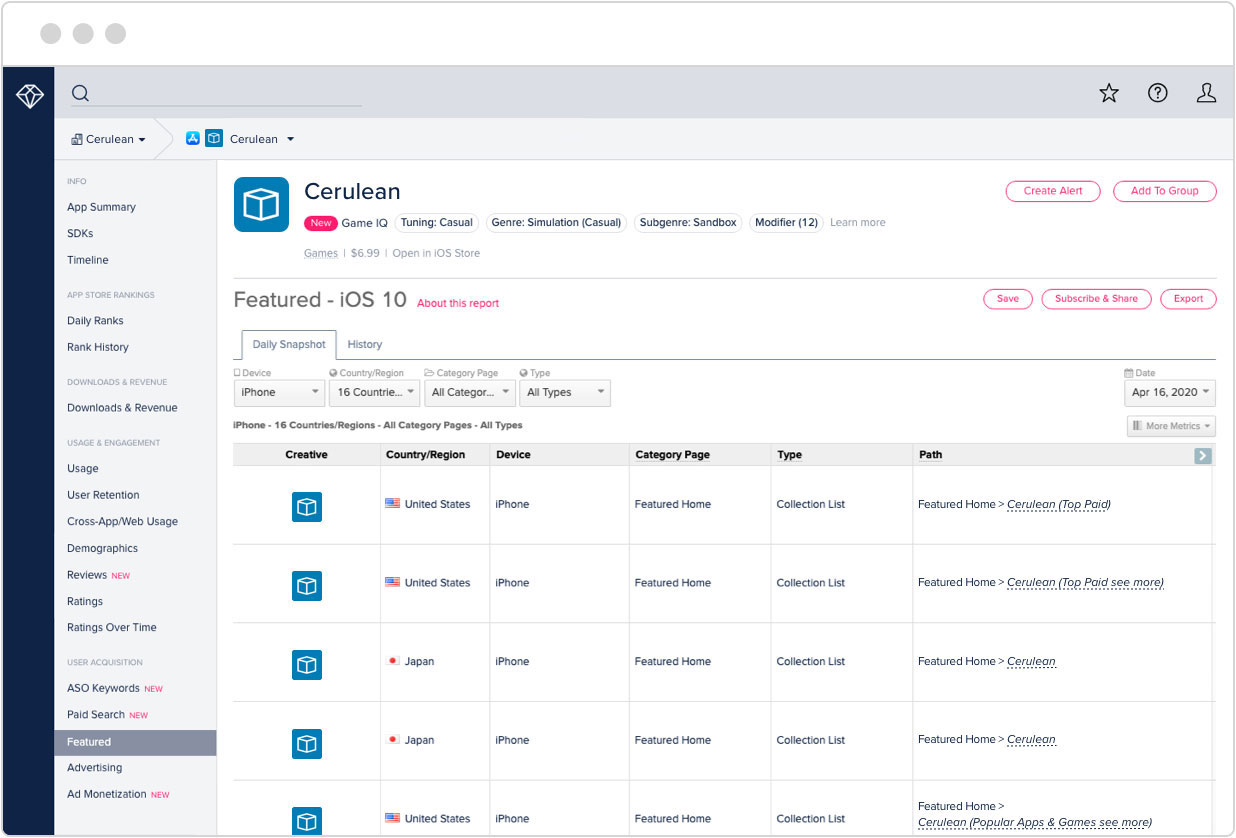# Mila's Tools - Unit Converter

### 免费注册来发掘更多！### 应用评价和评级### 应用说明

The award winning iPhone app makes its way to the Mac. Mila’s Tools is an elegant, no frills, fast, easy to use, unit conversion calculator. Included converters: Area, Bits & Bytes, Capacitance, Fraction, Fuel, Length, Liquid(Volume), Power, Pressure, Temperature, Time, Torque, Velocity and Weight. All built exclusively for Apple devices.

-------------------------------------

WINNER - Apple Staff Pick!

-------------------------------------

* 100+ CONVERSIONS
* FASTEST CONVERTER AVAILABLE
* NO DIALS
* AUTO CONVERTS MULTIPLE UNITS AS YOU TYPE

-------------------------------------

What customers are saying:

*****
Get it! Nothing better than this!

*****
This is the best quick ref tool I have come across to date

*****
Undoubtedly the best conversion app in the App Store.

*****
Best app for me so far.

*****
Intuitive interface. The app design is so easy you'll actually use it.

-------------------------------------

Full list of unit converters:

Area Converter
- square centimeters
- square meters
- hectares
- square kilometers
- square inches
- square feet
- square yards
- square miles
- acres

Bits & Bytes Converter
- bits (b)
- bytes (B)
- kilobits (kb)
- kilobytes (kB)
- megabits (Mb)
- megabyte (MB)
- gigabits (Gb)
- gigabytes (GB)
- terabits (Tb)
- terabytes (TB)
- petabytes (PB)
- exabytes (EB)
- zettabytes (ZB)

Blood Sugar Converter
- mg/dl
- mmol/l

Capacitance Converter

Fraction Converter
- Fraction
- Decimal
- Percent

Fuel Converter
- MPG (UK)
- MPG (US)
- miles/liter
- Km/liter
- Liters/100Km

Length Converter
- angstroms
- millimeters
- centimeters
- decimeter
- inches
- hands
- feet
- yards
- meters
- fathoms
- rods
- chains
- furlongs
- kilometers
- miles
- nautical miles
- leagues

Power Converter
- BTU/hour
- BTU/second
- calories/second
- erg/second
- foot pound/second
- gigawatt (GW)
- horsepower (hp)
- kilocalories/second
- kilojoules (kJ)
- kilowatt (kW)
- megawatt (MW)
- milliwatt (mW)
- watt (joules/sec)(W)

Pressure Converter
- lbs/ft^2
- mbar
- hPa
- kPa
- psi
- bar
- MPa

Temperature Converter
- celsius (c)
- fahrenheit (f)
- kelvin (k)
- rankine
- reaumur (r)

Time Converter
- microseconds
- milliseconds
- seconds
- minutes
- hours
- days
- weeks
- months
- years
- centuries

Torque Converter
- dyne centimeter
- gram centimeter
- kilogram centimeter
- kilogram meter
- kilonewton meter
- kilopond meter
- meganewton meter
- micronewton meter
- millinewton meter
- newton centimeter
- newton meter
- ounce foot
- ounce inch
- pound foot
- poundal foot
- pound inch

Velocity Converter
- feet per second (fps)
- feet per minute (fpm)
- meters per second (mps)
- miles per hour (mph)
- kilometers per hour (km/h)
- knots per hour (knots)

Volume Converter
- US teaspoons (tsp)
- US tablespoons (T)
- US ounces (fl oz)
- cups
- US pints (pt)
- US quarts (qt)
- US gallons (gal)
- US bushels (bu)
- beer barrels (bl)
- oil barrels (bbl)
- UK teaspoons (tsp)
- UK tablespoons (T)
- UK ounces (fl oz)
- UK pints (pt)
- UK quarts (qt)
- UK gallons (gal)
- UK bushels (bu)
- kilolitres (kL)
- litres (L)
- centilitres (cL)
- millilitres (mL)
- cubic inches
- cubic feet
- cubic yards
- cubic meters
- cubic centimeters

Weight Converter
- milligrams
- grains
- carats
- grams
- ounces
- troy ounces
- newtons
- pounds
- kilograms
- decanewtons
- stones
- metric tons
- short tons
- long tons